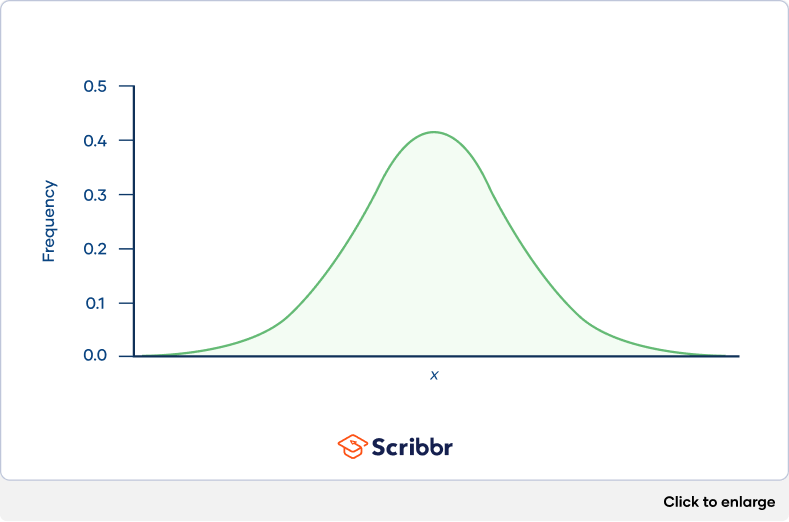# Skewness | Definition, Examples & Formula

Skewness is a measure of the asymmetry of a distribution. A distribution is asymmetrical when its left and right side are not mirror images.

A distribution can have right (or positive), left (or negative), or zero skewness. A right-skewed distribution is longer on the right side of its peak, and a left-skewed distribution is longer on the left side of its peak: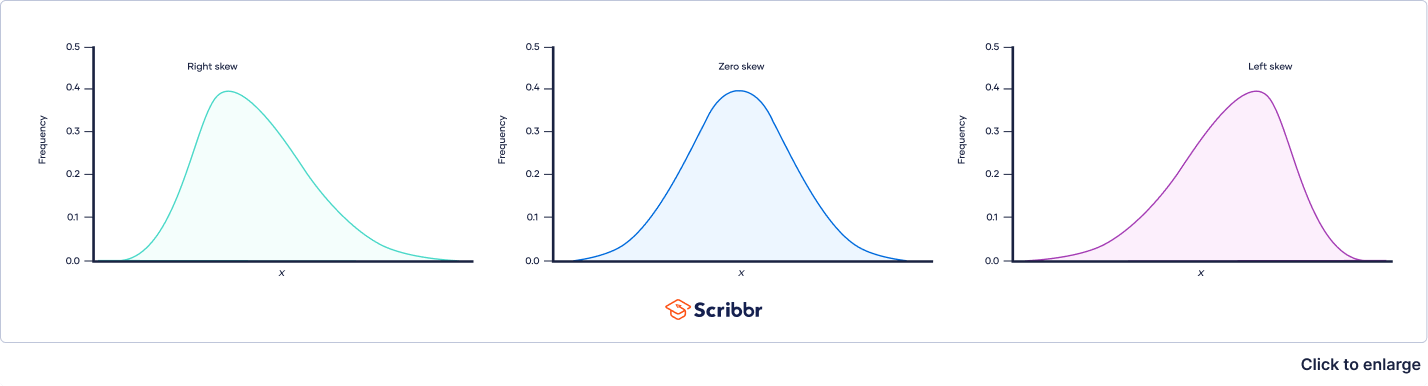You might want to calculate the skewness of a distribution to:

• Describe the distribution of a variable alongside other descriptive statistics
• Determine if a variable is normally distributed. A normal distribution has zero skew and is an assumption of many statistical procedures.

## What is zero skew?

When a distribution has zero skew, it is symmetrical. Its left and right sides are mirror images.

Normal distributions have zero skew, but they’re not the only distributions with zero skew. Any symmetrical distribution, such as a uniform distribution or some bimodal (two-peak) distributions, will also have zero skew.

The easiest way to check if a variable has a skewed distribution is to plot it in a histogram. For example, the weights of six-week-old chicks are shown in the histogram below.

The distribution is approximately symmetrical, with the observations distributed similarly on the left and right sides of its peak. Therefore, the distribution has approximately zero skew.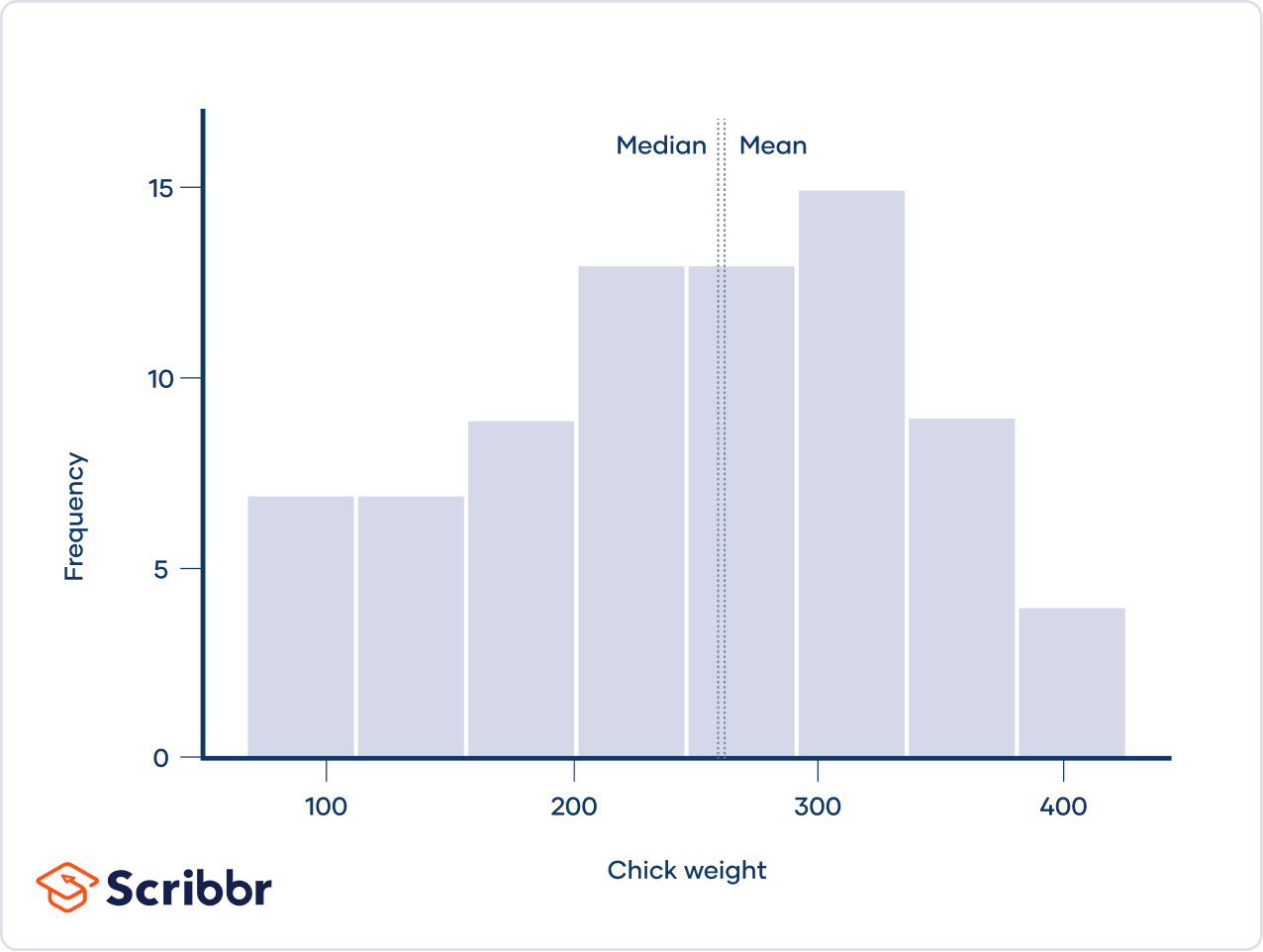In a distribution with zero skew, the mean and median are equal.

For example, the mean chick weight is 261.3 g, and the median is 258 g. The mean and median are almost equal. They aren’t perfectly equal because the sample distribution has a very small skew.

Although a theoretical distribution (e.g., the z distribution) can have zero skew, real data almost always have at least a bit of skew. However, if a distribution is close to being symmetrical, it usually is considered to have zero skew for practical purposes, such as verifying model assumptions.

## What is right skew (positive skew)?

A right-skewed distribution is longer on the right side of its peak than on its left. Right skew is also referred to as positive skew.

You can think of skewness in terms of tails. A tail is a long, tapering end of a distribution. It indicates that there are observations at one of the extreme ends of the distribution, but that they’re relatively infrequent. A right-skewed distribution has a long tail on its right side.

The number of sunspots observed per year, shown in the histogram below, is an example of a right-skewed distribution. The sunspots, which are dark, cooler areas on the surface of the sun, were observed by astronomers between 1749 and 1983.

The distribution is right-skewed because it’s longer on the right side of its peak. There is a long tail on the right, meaning that every few decades there is a year when the number of sunspots observed is a lot higher than average.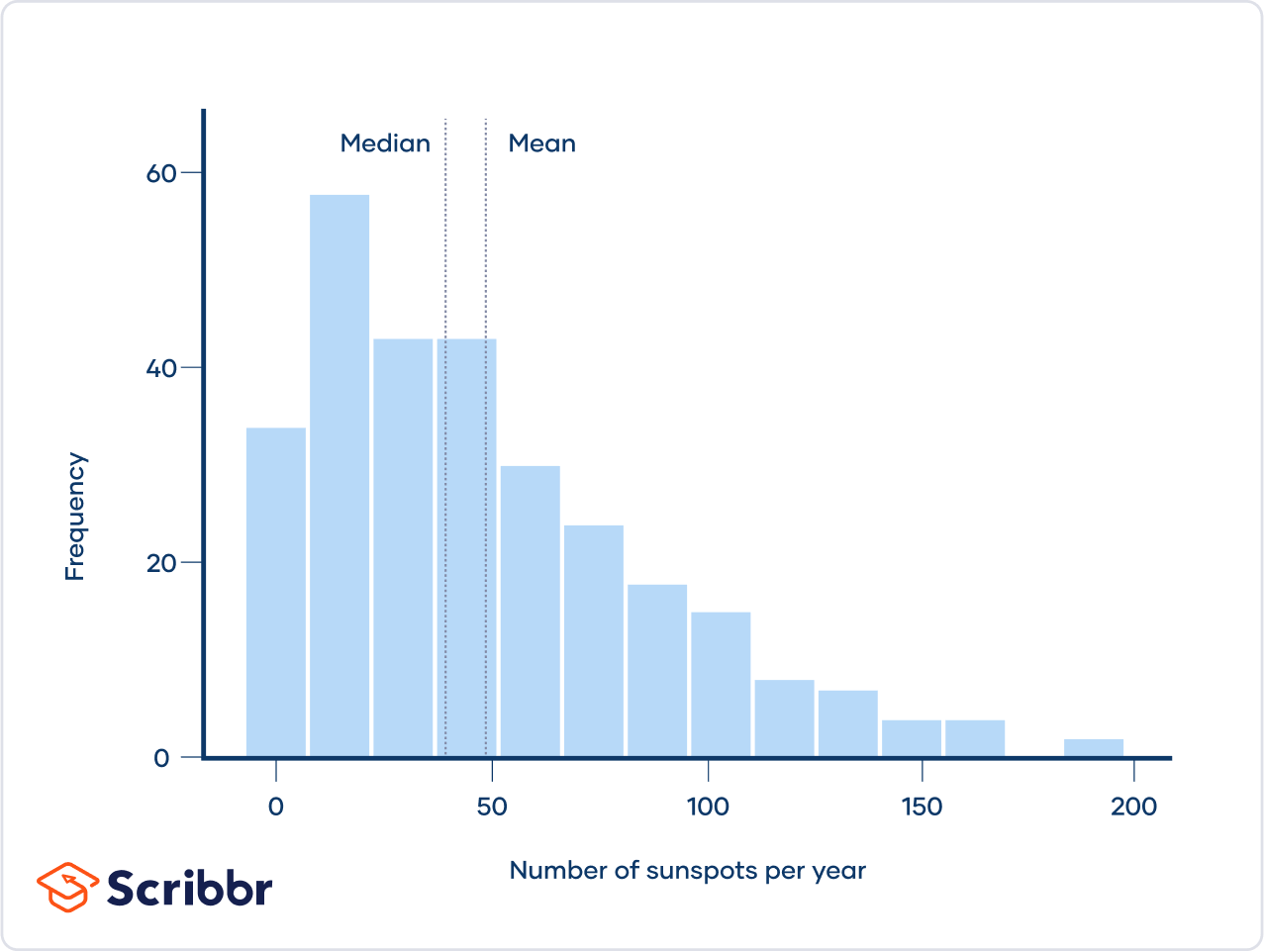The mean of a right-skewed distribution is almost always greater than its median. That’s because extreme values (the values in the tail) affect the mean more than the median.

For example, the mean number of sunspots observed per year was 48.6, which is greater than the median of 39.

## What is left skew (negative skew)?

A left-skewed distribution is longer on the left side of its peak than on its right. In other words, a left-skewed distribution has a long tail on its left side. Left skew is also referred to as negative skew.

Test scores often follow a left-skewed distribution, with most students performing relatively well and a few students performing far below average. The histogram below shows scores for the zoology portion of a standardized test taken by Indian students at the end of high school.

The distribution is left-skewed because it’s longer on the left side of its peak. The long tail on its left represents the small proportion of students who received very low scores.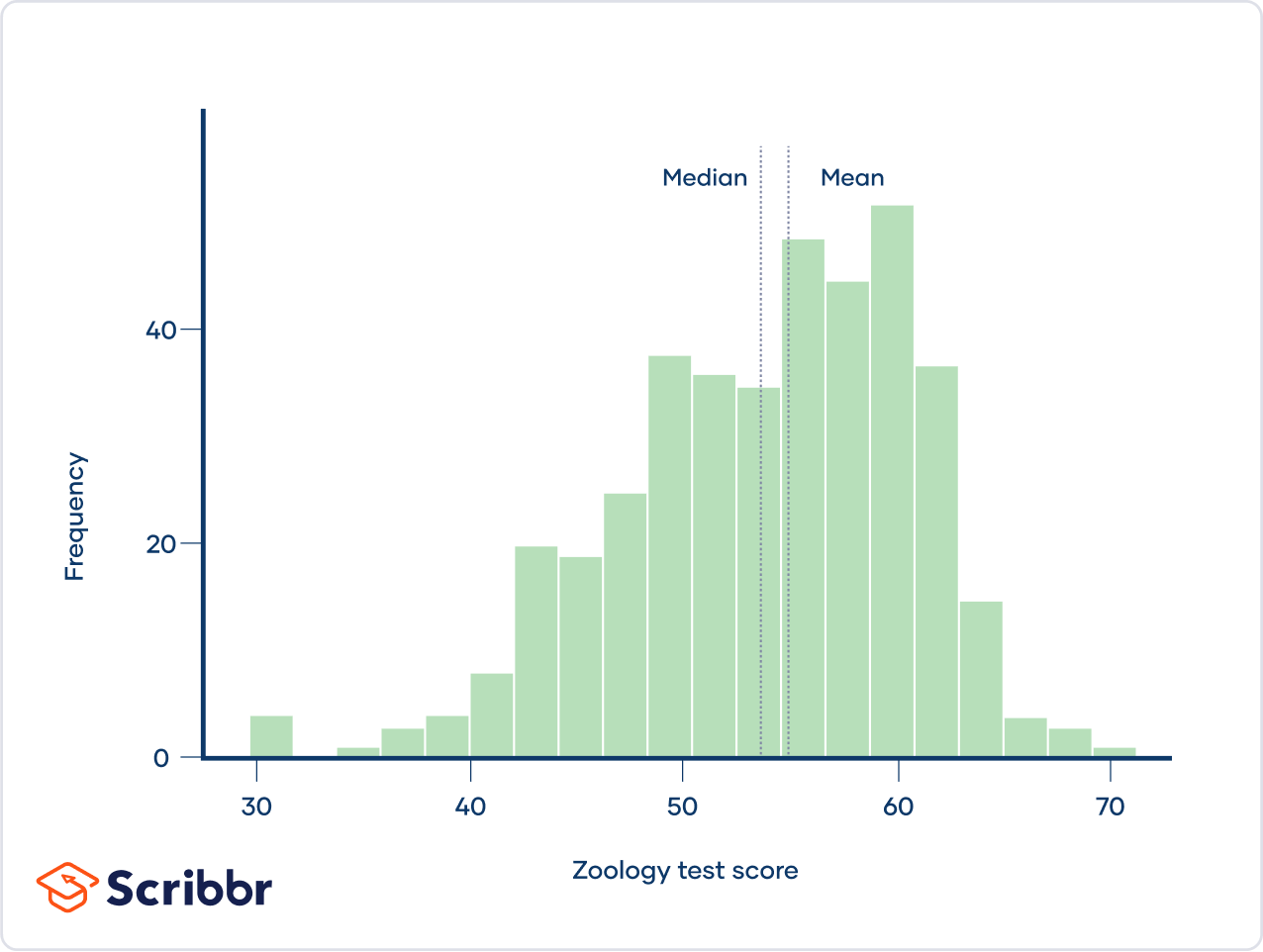The mean of a left-skewed distribution is almost always less than its median.

For example, the mean zoology test score was 53.7, which is less than the median of 55.

## How to calculate skewness

There are several formulas to measure skewness. One of the simplest is Pearson’s median skewness. It takes advantage of the fact that the mean and median are unequal in a skewed distribution.

Pearson’s median skewness tells you how many standard deviations separate the mean and median.

Real observations rarely have a Pearson’s median skewness of exactly 0. If your data has a value close to 0, you can consider it to have zero skew. There’s no standard convention for what counts as “close enough” to 0 (although this research suggests that 0.4 and −0.4 are reasonable cutoffs for large samples).

## What to do if your data is skewed

One reason you might check if a distribution is skewed is to verify whether your data is appropriate for a certain statistical procedure. Many statistical procedures assume that variables or residuals are normally distributed. Skew is a common way that a distribution can differ from a normal distribution.

You generally have three choices if your statistical procedure requires a normal distribution and your data is skewed:

1. Do nothing. Many statistical tests, including t tests, ANOVAs, and linear regressions, aren’t very sensitive to skewed data. Especially if the skew is mild or moderate, it may be best to ignore it.
1. Use a different model. You may want to choose a model that doesn’t assume a normal distribution. Non-parametric tests or generalized linear models could be more appropriate for your data.
1. Transform the variable. Another option is to transform a skewed variable so that it’s less skewed. “Transform” means to apply the same function to all the observations of a variable.
 Type of skew Intensity of skew Transformation Right Mild Do not transform Moderate Square root Strong Natural log Very strong Log base 10 Left Mild Do not transform Moderate Reflect* then square root Strong Reflect* then natural log Very strong Reflect* then log base 10

*In this context, “reflect” means to take the largest observation, K, then subtract each observation from K + 1. Keep in mind that the reflection reverses the direction of the variable and its relationships with other variables (i.e., positive relationships become negative).

## Practice questions

What is the difference between skewness and kurtosis?

Skewness and kurtosis are both important measures of a distribution’s shape.

• Skewness measures the asymmetry of a distribution.
• Kurtosis measures the heaviness of a distribution’s tails relative to a normal distribution.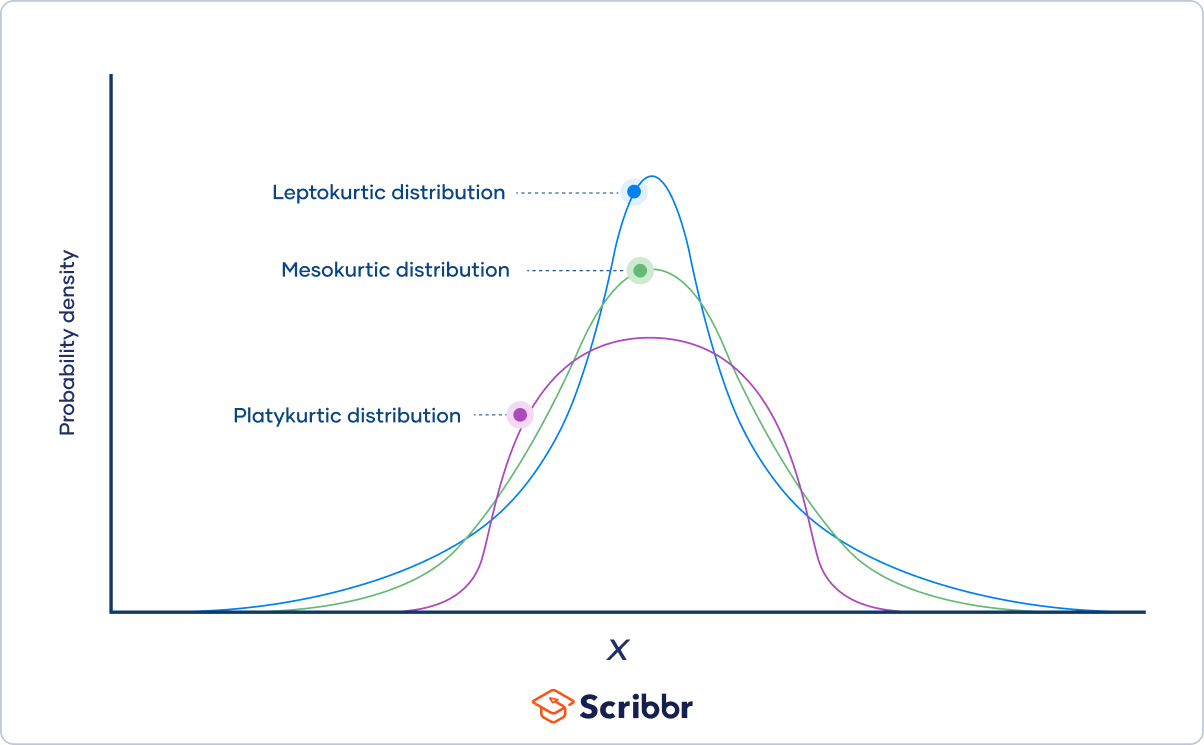What are the three types of skewness?

The three types of skewness are:

• Right skew (also called positive skew). A right-skewed distribution is longer on the right side of its peak than on its left.
• Left skew (also called negative skew). A left-skewed distribution is longer on the left side of its peak than on its right.
• Zero skew. It is symmetrical and its left and right sides are mirror images.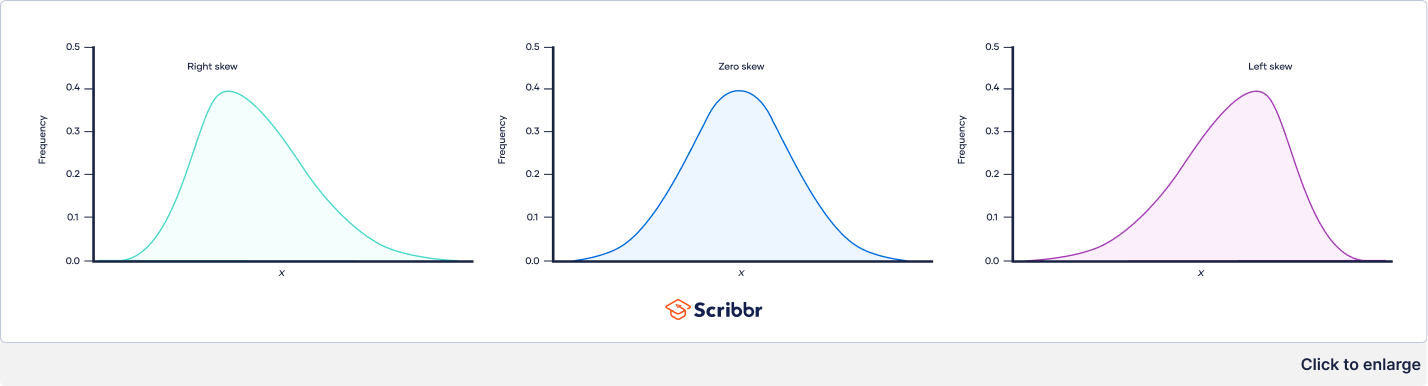What is a normal distribution?

In a normal distribution, data are symmetrically distributed with no skew. Most values cluster around a central region, with values tapering off as they go further away from the center.

The measures of central tendency (mean, mode, and median) are exactly the same in a normal distribution.